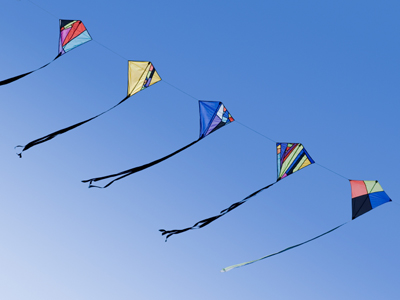You'll find a few kites in this quiz!

# Shapes 8

This Math quiz is called 'Shapes 8' and it has been written by teachers to help you if you are studying the subject at middle school. Playing educational quizzes is a fabulous way to learn if you are in the 6th, 7th or 8th grade - aged 11 to 14.

It costs only \$12.50 per month to play this quiz and over 3,500 others that help you with your school work. You can subscribe on the page at Join Us

In this quiz about shapes we are going to look at quadrilaterals: these are closed 2D (two dimensional) shapes: being 2D they are flat and have no volume.

The word 'quadrilateral' comes from two Latin words, 'quadri' and 'latus'. Quadri means four and latus means side. Think of a quad bike - why is it called this? Because it has four wheels. If you hear of someone giving birth to 'quads', you'll now know how many babies there would be.

1.
Which of the following shapes is also a parallelogram?
Kite
Rhombus
Trapezoid
Trapezium
The square, rhombus and rectangle are also parallelograms because their opposite sides are parallel and equal in length AND their opposite angles are equal in size
2.
What is the name given to a quadrilateral whose sides are all the same length and whose interior angles are all the same size?
The term 'regular' is also used for polygons whose side lengths are all the same, e.g. a regular pentagon has five sides all of the same length, and its interior angles are all the same size: 108°
3.
What is the name of the quadrilateral that has the following properties?
It has diagonally opposite equal angles.
Its opposite sides are of the same length.
Its opposite sides are parallel.
Its diagonals bisect each other.
Rectangle
Rhombus
Parallelogram
Kite
Learn these facts
4.
There is only one regular quadrilateral. What is it called?
A rectangle
A parallelogram
A square
A rhombus
That's a fact worth knowing
5.
What is the name of the quadrilateral that has the following properties?
It has two pairs of sides of equal length.
Only one pair of diagonally opposite angles is equal.
Only one of its diagonals is bisected by the other.
Its diagonals intersect at 90°.
Kite
Rhombus
Parallelogram
Square
Learn these facts
6.
What is the name of the quadrilateral that has the following properties?
Its diagonally opposite angles are the same.
Its sides are of equal length.
Its opposite sides are parallel.
Its diagonals bisect each other at 90°.
Parallelogram
Rhombus
Kite
Trapezium
Learn these facts
7.
What is the name of the quadrilateral that has the following properties?
All its interior angles are 90°.
Its opposite sides are of the same length.
Its diagonals are of the same length.
Parallelogram
Rhombus
Square
Rectangle
A 'rectangle' is also called an 'oblong'. Learn these facts
8.
What is the name of the quadrilateral that has the following property?
Only one pair of opposite sides is parallel.
Trapezium
Rhombus
Square
Kite
A 'trapezoid' is like a trapezium, but it doesn't have any parallel sides. The words 'trapezium' and 'trapezoid' have been used as defined in British English. Learn these facts
9.
What is the sum of the interior angles of a quadrilateral?
180°
720°
360°
270°
A quadrilateral can be split into two non-overlapping triangles: 180° + 180° = 360°
10.
What is the name of the quadrilateral that has the following properties?
All its interior angles are 90°.
All its sides are of equal length.
Its opposite sides are parallel.
Its diagonals bisect each other at 90°.
Its diagonals are of equal length.
Trapezium
Rectangle
Rhombus
Square
Learn these facts
Author:  Frank Evans